# OpenCV-Python轮廓特征

• 查找轮廓的不同特征，例如面积，周长，质心，边界框等
• 您将看到大量与轮廓有关的功能。

### OpenCV轮廓特征-矩

import numpy as np
import cv2 as cv
ret,thresh = cv.threshold(img,127,255,0)
contours,hierarchy = cv.findContours(thresh, 1, 2)
cnt = contours
M = cv.moments(cnt)
print( M )


cx = int(M['m10']/M['m00'])
cy = int(M['m01']/M['m00'])


### OpenCV轮廓面积

area = cv.contourArea(cnt)


### OpenCV轮廓周长

perimeter = cv.arcLength(cnt,True)


### OpenCV轮廓近似

epsilon = 0.1*cv.arcLength(cnt,True)
approx = cv.approxPolyDP(cnt,epsilon,True)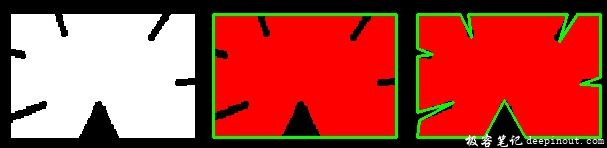### OpenCV凸包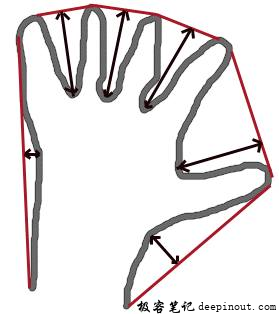hull = cv.convexHull(points[, hull[, clockwise[, returnPoints]]])


• points: 就是我们传入的轮廓。
• hull: 是输出，通常我们避免它。
• clockwise：方向标记。如果为True，则输出凸包为顺时针方向。否则，其方向为逆时针方向。
• returnPoints：默认情况下为True。然后返回船体点的坐标。如果为False，则返回与船体点相对应的轮廓点的索引。

hull = cv.convexHull(cnt)


### OpenCV检查凸度

cv.isContourConvex() 是一个函数用来检查曲线是否为凸多边形。它只是返回True还是False。

k = cv.isContourConvex(cnt)


### OpenCV边界矩形

#### a. OpenCV直角矩形

x,y,w,h = cv.boundingRect(cnt)
cv.rectangle(img,(x,y),(x+w,y+h),(0,255,0),2)


#### b．OpenCV旋转矩形

rect = cv.minAreaRect(cnt)
box = cv.boxPoints(rect)
box = np.int0(box)
cv.drawContours(img,[box],0,(0,0,255),2)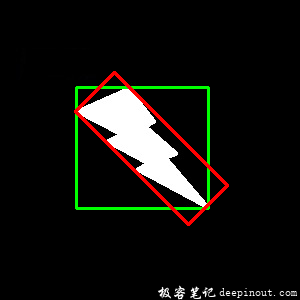### OpenCV最小外圆

(x,y),radius = cv.minEnclosingCircle(cnt)
center = (int(x),int(y))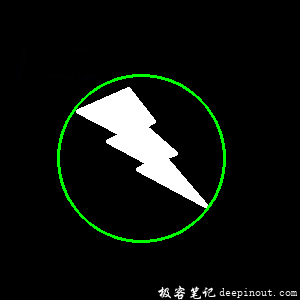### OpenCV拟合椭圆

ellipse = cv.fitEllipse(cnt)
cv.ellipse(img,ellipse,(0,255,0),2)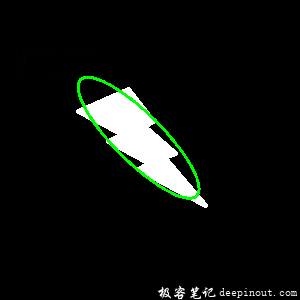### OpenCV拟合线

rows,cols = img.shape[:2]
[vx,vy,x,y] = cv.fitLine(cnt, cv.DIST_L2,0,0.01,0.01)
lefty = int((-x*vy/vx) + y)
righty = int(((cols-x)*vy/vx)+y)
cv.line(img,(cols-1,righty),(0,lefty),(0,255,0),2)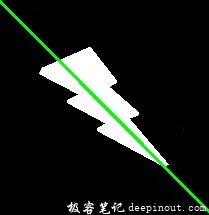• 回顶